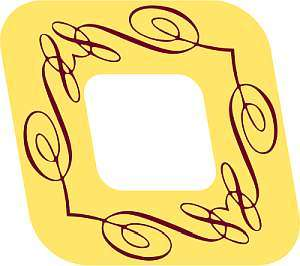# Area of a Rhombus

Instructions: Use this calculator to find the area of a rhombus, with a given diagonals p and q. Please provide the diagonals in the form box below.One diagonal $$p$$ of the rhombus (Ex: 2, or 3/2, etc.)The other diagonal $$q$$ of the rhombus (Ex: 2, or 3/2, etc.)

This calculator will compute for you the area of a rhombus, for the two diagonals of the rhombus that you provide. The provided diagonals must valid numeric expressions. You can type any valid expressions, included numbers like '4' or '3.56', or you algebraic expressions like '2/3', or 'sqrt(3)' as well.

All you need to do is to provide the two valid expressions that represent the diagonals, and then click on "Calculate" button, and all the steps of the calculation will be shown.

The calculation of the area of the rhombus is a perhaps less common task, at least when compared with the computation of the area of a rectangle and the area of a square. Still, the calculation of geometric areas is a crucial skill learned in Algebra.## How do compute the area of a rhombus?

Using a symmetry consideration, the calculation of the area of the rhombus is quite similar to what you would do to compute the area of a triangle. The formula for the area of a rectangle is

$\text{Area} = \displaystyle \frac{p q}{2}$

where p and q correspond to the respective diagonals

## What are the steps for computing the area of a rhombus

• Step 1: Identify the diagonals of them rhombus that are being provided, and call them 'p' and 'q'
• Step 2: Once have the diagonals 'p' and 'q' identified, the area is computed as p*q/2
• Step 3: This means that to get the area, you multiply the diagonals and the result of that multiplication is divided by 2
• Step 4: If needed, identify the units of 'p' and 'q' (if any) and give units to the area

## Are there any applications of the rhombus?

Certainly All geometric shapes usually can be used in many different way. For example, the shape of a baseball field is (ideally) of the shape of a perfect rhombus, but that is one example out of many.### Example: Calculate the area of a rhombus

Calculate the area of rhombus with given diagonals equal to p = 3 and q = 4.

Solution: The step consists of precisely identifying the diagonals. In this, it is clearly stated that the diagonals are p = 3 and q = 4. The formula for the area that needs to be used is:

$Area = \displaystyle \frac{pq}{2}$

Now, by plugging the values of p = 3 and q = 4 into the formula we get:

$Area = \displaystyle \frac{pq}{2} = \displaystyle \frac{3 \times 4}{2} = \displaystyle \frac{12}{2} = 6$

so the area is 6.

### Example: Area of a rectangle with units

Calculate the area of rhombus with diagonals p = 2.2 cm and q = 6.1 cm

Solution: Like in the previous example, the first task corresponds to identifying the diagonals, which in this case are p = 2.2 cm and q = 6.1 cm, and in this example the diagonals come with length units. The area is:

$Area = \displaystyle \frac{pq}{2}$

Now, by plugging a = 2.2 cm and b = 6.1 cm into the formula, we get that:

$Area = \displaystyle \frac{pq}{2} = \displaystyle \frac{2.2 \times 6.1}{2} cm^2 = 13.42 cm^2$

which indicates that the area is 13.42 cm2.

## More area calculators

Geometric shapes usually have simple formulas for the calculation of areas. The calculation of the area of a rhombus and the area of a triangle are considered basic skills in Algebra and Geometry..

Also, naturally other kind of symmetric shapes have a lot of interest, and certainly you may like to take a look at our area of circle calculator and our area of an ellipse calculator.